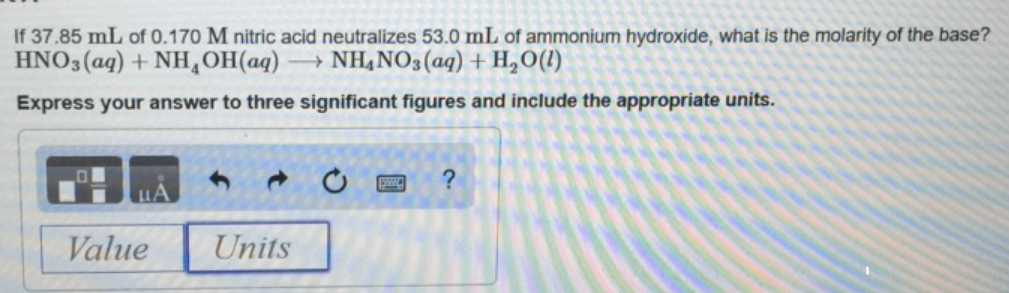# Problem: If 37.85 mL of 0.170 M nitric acid neutralizes 53.0 mL of ammonia, what is the molarity of the base?HNO3 (aq) + NH4OH (aq) → NH4 NO3 (aq) + H2O(l)Express your answer to three significant figures and include the appropriate units.

###### FREE Expert Solution
79% (85 ratings)###### Problem Details

If 37.85 mL of 0.170 M nitric acid neutralizes 53.0 mL of ammonia, what is the molarity of the base?

HNO3 (aq) + NH4OH (aq) → NH4 NO3 (aq) + H2O(l)

Express your answer to three significant figures and include the appropriate units.